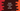# How to compare two strings in Kotlin in different ways## Different ways in Kotlin to compare two strings:

This post will show you how to compare two strings in Kotlin with examples. There are different ways to compare two strings. Let’s learn them one by one.

### Method 1: Compare two string by using the comparison operator == in Kotlin:

Let’s try with the comparison operator, == to check if two strings are equal or not. It returns true if two strings are equal structurally.

``````fun main() {
val str1 = "hello"
val str2 = "hello"
val str3 = "Hello"

println(str1 == str2)
println(str2 == str3)
}``````

The content of str1 and str2 are equal but str3 is different. If you run this program, it will print:

``````true
false``````

The first comparison prints true because the content of str1 and str2 are equal. The second comparison prints false because the content of str2 and str3 are different.

We can also use === to compare two strings but it is used to check for reference equality i.e. if both string variables are pointing to the same object or not.

``````fun main() {
val str1 = "hello"
val str2 = "hello"
val str3 = String("hello".toCharArray())

println(str1 === str2)
println(str2 === str3)
}``````

Here, str1 and str2 are pointing to the same object in memory. But str2 and str3 are pointing to different objects. So, the first print statement will print true and the second print statement will print false.

If we use == in the above example, it will print true for both.

### Method 2: Compare two string by using the equals method in Kotlin:

We can also use the equals method to compare two strings in Kotlin. This method is similar to the == operator. It is defined as like below:

``public actual fun String?.equals(other: String?, ignoreCase: Boolean = false): Boolean``

The second parameter, ignoreCase is a boolean parameter and it is optional. If you pass true, it will ignore the character case while comparing the strings. It returns a boolean value.

Let’s try it with one example:

``````fun main() {
val str1 = "hello"
val str2 = "hello"
val str3 = String("hello".toCharArray())

println(str1.equals(str2))
println(str2.equals(str3))
}``````

The equals method is similar to ==. If you run the above program, it will print true for both.

``````true
true``````

This is because it is comparing the values, not their references. The advantage of equals method is that we can pass the second parameter ignoreCase as true to ignore character case while comparing two strings.

``````fun main() {
val str1 = "hello"
val str2 = "HELLO"

println(str1.equals(str2, ignoreCase = true))
}``````

It will print true as we marked ignoreCase as true.

### Method 3: Compare two strings by using the compareTo method:

The compareTo function actually checks the order of two strings. It returns one integer value. This method is defined as like below:

``str1.compareTo(str2: string, ignoreCase: Boolean = false): Int``

It compares the string str1 and str2 for order. It returns one integer value.

• 0 if both str1 and str2 are equal.
• A negative value if str1 is less than str2.
• A positive value if str1 is greater than str2.

The second argument ignoreCase is an optional parameter. If we pass true, it will ignore character case while comparing the strings.

Let’s take a look at the below example:

``````fun main() {
val str1 = "hello"
val str2 = "HELLO"
val str3 = String("hello".toCharArray())

println(str1.compareTo(str2))
println(str1.compareTo(str3))
println(str1.compareTo(str2, ignoreCase = true))
}``````

It will print:

``````32
0
0``````

The second statement prints 0 because str1 and str3 are equal if we ignore character case. The third statement prints 0 because the contents of str1 and str2 are equal.